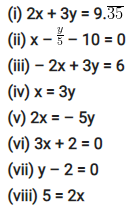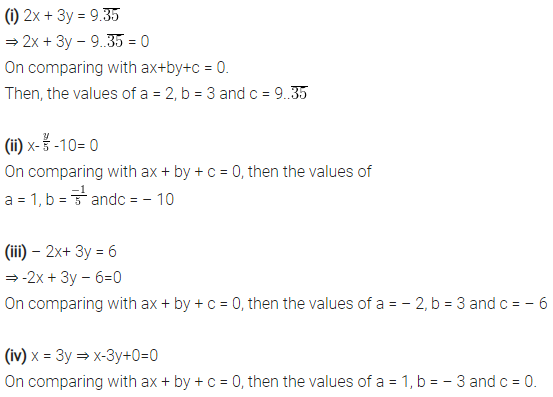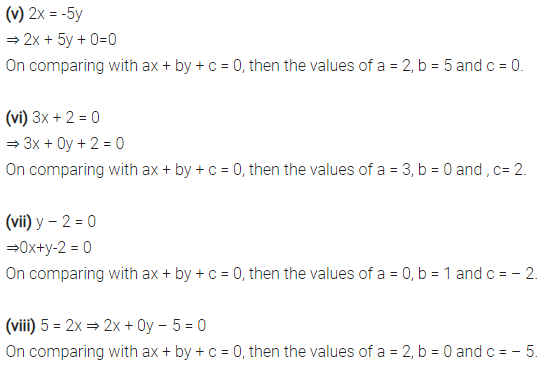# Express the following linear equations in the form ax + by + c = 0

Express the following linear equations in the form ax + by + c = 0 and indicate the values of a, b and c in each case.